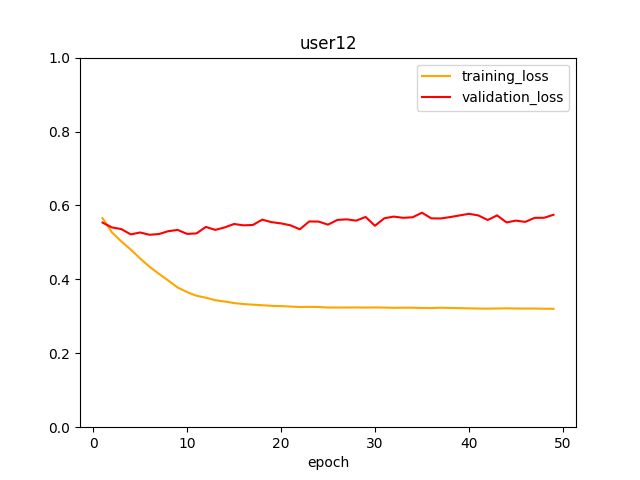# CrossentropyLoss with Softmax?

Hi there,

I am recently moved from keras to pytorch. I am trying to train a model for a classification problem. I am aiming to use transfer learning. I used Googlenet architecture and add custom layer below it. Also I am using CrossEntropyLoss() for criterion.

``````model = torchvision.models.googlenet(True)
# Customizing fc layers of the model
model.fc = nn.Sequential(
nn.Linear(1024, 2),
nn.Softmax() #Question here!!
)
criterion = nn.CrossEntropyLoss()
``````

As in the stated 1.9.0 docs CrossEntropyLoss combines `LogSoftmax` and `NLLLoss` in one single class. However, when it comes to the 1.11 docs this information is gone.

My question is that for such a 2-class classification problem, should I add softmax layer or not?

I tried both for sure, before writing this questions. When I add softmax explicitly, preferable results are shown, however training loss never converges to zero;However, When I am not adding the softmax;

``````model = torchvision.models.googlenet(True)
# Customizing fc layers of the model
model.fc = nn.Sequential(
nn.Linear(1024, 2),
# nn.Softmax() #Question here!!
)
criterion = nn.CrossEntropyLoss()
``````

Training losses converges really quick (almost linearly), but validation loss starts to increase; (I am aware that second example includes only 10 epochs, but this information should be enough to compare.)Model with the softmax Seems not much over-fitting and Not OK but at least better results.

There are several questions on the forum about transfer learning and no one added softmax layer, even the official tutorial. What is the correct way of transfer learning for classification? Should I add softmax? And When I add softmax layer, is it trained?

No, as `nn.CrossEntropyLoss` will still use `F.log_softmax` and `nn.NLLLoss` internally.

But how can you explain the results? Validation loss not even close. Or what do you suggest?

The additional and unneeded `softmax` will squeeze the logits to `[0, 1]` and thus also lower the gradient magnitude etc. so maybe your training could benefit from a lower learning rate?

Oh this explains my situation, thank you!

I am now trying to lower my learning rate. Can I ask you lasly, When I use “unneeded softmax”, why is it prevents training loss to be converged?

I would think for the same reason of lowering the output and thus also the gradient magnitudes, which might cause your model training to get stuck.
Note that this is just pure speculation so maybe someone else has a more mathematical explanation.

Thank you! My problem is now evolved into overfitting I guess.I think as you said

`nn.CrossEntropyLoss` will still use `F.log_softmax` and `nn.NLLLoss` internally

CrossEntropyLoss combines `LogSoftmax` and `NLLLoss` in one single class

should be back to the documentation.

Thanks for help again!

It it still there, but now split into two cases.
In newer PyTorch version you are able to use “soft” targets (i.e. probabilities instead of class indices) as the target tensor so the documentation was split into:

``````...
The target that this criterion expects should contain either:

1) Class indices in the range ...
Note that this case is equivalent to the combination of LogSoftmax and NLLLoss.

2) Probabilities for each class;
...
``````

and the first case still equals to the `LogSoftmax` and `NLLLoss` case.